.Force of Gravity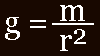The reason why Newton's theory on the force of gravity calculates as g=m/r^2 in 3-dimensional space can be seen in this image:As the distance from the planet is doubled, so the force of gravity must spread out over four times the area. This is why r is to the power 2. (It squares). If the universe were two-dimensional (a flat plane), then, when the distance from the body doubled, the force of gravity would spread out over twice the space. (r would not be squared)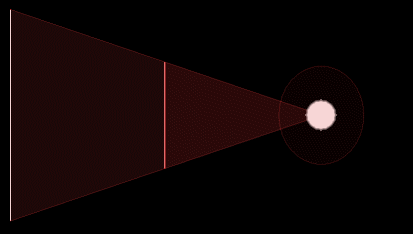But as our universe is three-dimensional, the force of gravity decreases by nine times when we are three times further away. This is why the variable ‘r’ is the denominator (at the bottom) and is squared: Because the force of gravity must be divided and get smaller exponentially the further away we get from the source of the gravity.But we take it for granted the distribution is perfect in either system. Why could gravity not be something like g=m/r^2.0000000000001 ? Such would constitute a difference of a thousandth of a mm for every light year. We would never notice it. But we take it on faith that the fraction is not there. We just know it is not so.

 The following articles are quick, and easy-to-read: Proof for instant gravity Or do you assume that popular opinion trumps geometry? Special Relativity orbits The affect of the limit at the velocity of light on an eccentric orbit. General Relativity orbits How an orbit would be shaped if gravity moves at lightspeed. Triple Orbit Software Is this the only algorithm which has computed 3 gravity fields? What is the speed of gravity? Including cross references to Hawking and LIGO

 This article and free algorithm examines the effect of Relativity on the Solar System and beyond. Most specifically it analyzes t how gravity moving at the speed of light effects the Alpha Centauri binary orbit. Various values for the gravitational constant are applied with the precision of just one millimeter to planetary orbits: Gravity and the orbits of the solar system

 Analysis of gravitational wave experiment GW150914 The surprising results of the algorithm orbit-gravity-sim-11.exe have to be observed in their actual real-time evolution to be appreciated. Essentially this proves that gravity must be instantaneous or else binary systems will out-spiral prodigiously. And yet the LIGO experiments claim precisely the opposite. This paradox is resolved in a way which was completely unexpected.

 Gravitational Waves & General Relativity A computational analysis of the theory of gravitational waves; as expressed within Einstein’s Theory of General Relativity and the wider realm of Astrophysics. Essentially a critique, this study has been written for the purpose of explaining the unobvious challenges faced in building graphically dynamic evolutionary computer models. These models compute the theoretical functionality of gravitational waves in the celestial paradigms of solar system formation and galaxy formation.

 The likelihood of circular orbits, given gravity and chance, are much the same regardless of the gravitational exponent. Even near-circular orbits are rare. Consider how much less like a solar system this would be if it were in three dimensions. Here are many bodies programmed using g=m/r^2, but with random starting points and no force of spin: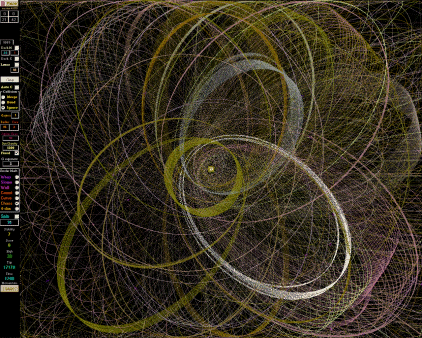What this shows is that the very essence of design, of form, of the universe itself, is intrinsically conscious; because without consciousness there can be no design, for consciousness is a necessary feature of design. As the Universe is clearly designed, consciousness must be at the root of it. This is termed Teleology. That the physical universe has an end point, a goal, and that all the laws of nature, known and unknown, are deliberately directed towards this final construct.

 This animation shows that in order for a solar system to form, the planets must have started from a position of spin or else the planets in the solar system would not have uniform orbits. A star already in a uniform orbit going nova dictates the starting positions of the planets.More on solar system formation here: Solar System Formation The software used to generate this animation is at these links: OG6: orbit-game-6.exe  OG7: orbit-game-7.exe

 A similar program was used to demonstrate how and why the Moon slowly recedes from the Earth. The gravity of the Sun is gently tugging on the Moon at 4cm per year.More on why the moon recedes from the Earth here: Moon Receding from Earth To download this program and watch in real time, click here: orbit-game-5.exe

 Another program was constructed to demonstrate the solution to Rubin's problem and the obscure nature of rotation curves of Spiral Galaxies.More information on rotation curves of Spiral Galaxies here: Rubin's Problem & Rotation Curves of Galaxies & Rubin's Problem Animated & Oscillations in Rotation Curves of Spiral Galaxies & Summary of Rotation Curves of Galaxies, describes the nature of how dark matter does not affect Newton's law of gravity To observe this process in real time, download here: orbit-game-4.exe or orbit-game-4-2.exe which has variables you can tweak. orbit-game-4-3.exe has more detail showing the binary pair separating, which is why more recent stars are emitted more slowly, and older stars more rapidly.

 This software deals with the shapes of Binary Orbits.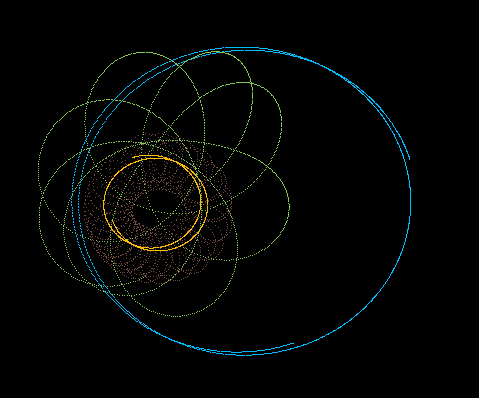To observe this process in real time, download here: orbit-game-8.exe.

 This page details the Big Unwind (previously called the 'Big Bang')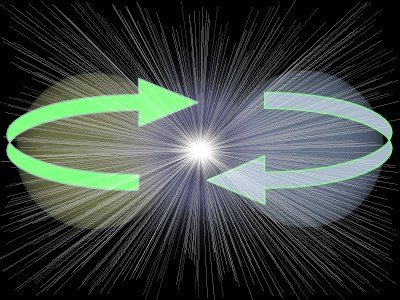To observe an animation of this process in real time, click here: Big Unwind

 In order to generate this software, some other questions needed to be answered: Xeno's Paradox, which deals with the math of Max Planck and quantum time, Quantum Gravity, which explains the slingshot affect, and The Many-Body-Problem, which opens a myriad of other possibilities.

 As a consequence of this software some fundamental questions were answered: Dark Matter was solved due to answering the question on rotation curves of Spiral Galaxies. Dark Energy was solved when applying all these calculations to the nature of how the Big Bang unwound. Gravitational Lensing & Binary Orbits & Gravity Simulators & Relativity Revised Einstein's errors have been corrected. .

 How birds and other flying phenomenon defy gravity: Principles of FlightThe undeniable point being that in order to get anything to work properly, regardless of whether it is a solar system, galaxy, life-form, or flying machine, it would need to be deliberately and perfectly designed to do so. But a more subtle point implied in this, is that we need to have Cartesian Dualism as an intrinsic and fundamental feature of the system in question. Design implies that the perspective of design stands outside from that which is being designed. The blueprint for the design of a house cannot be part of the house itself. The blueprint must be external to the house. Similarly, if the blueprint of an object were intrinsically and completely inside the object being designed it would need to have existed in its pure form before it manifested in its physical form, which means that its true form would have to be a priori to its physical form. Thus the underlying reality of the physical world is a construct like the shadows on the wall of Plato’s cave.

 If this is all a bit much to try and absorb at once, it would be s good idea to buy the book. These ideas are better discussed in the logical progression outlayed in print format. There are many more diagrams and countless new designs for weird and wonderful aircraft in the book.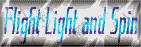< top >

< seti >

< why? >

< credits >

< gravity >

< dark matter >

< dark energy >

< binary orbits >

< the big unwind >

< contact details >

< zeno and planck >

< centrifugal force >

< quantum gravity >

< relativity revised >

< principle of flight >

< gravity simulators >

< 3d printer projects >

< entothopter videos >

< free fractal software >

< why is the sky blue? >

< entothopter project >

< gravitational lensing >

< sketchup entothopter >

< ezekiel's flying machine >

< the many-body problem >

< philosophy of computers >

< genealogy and exponents >

< bin laden helicopter crash >

< how the solar system formed >

< gravitational waves & general relativity >

< why the moon is receding from the earth >

< why the moon is red during a lunar eclipse >

< rubin's problem - rotation curves of galaxies - rubin's problem animated - oscillations in spiral galaxies >